Arduino 制作一个音乐键盘

1. 发声的问题

2. USB 键盘数据的解析；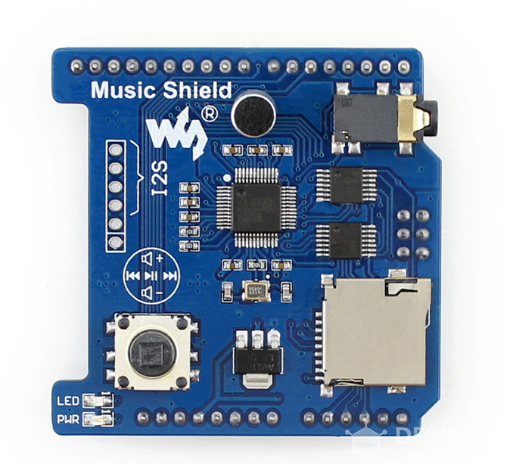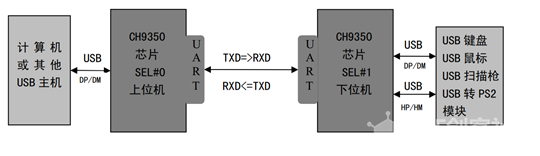1x
CH9350 Mini 模块
1x

1x
Arduino Proto Shield (用于固定 CH9350 Mini，无任何元件)
1x
Arduino Proto Shield 扩展（用于插接 Music Shield,无任何元件）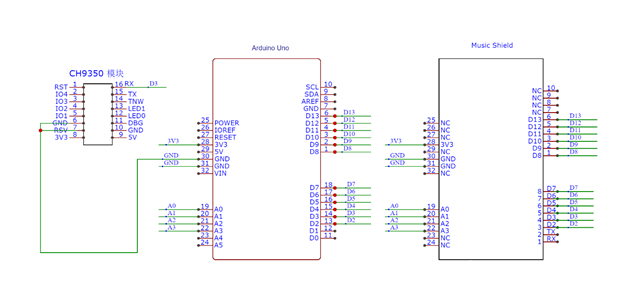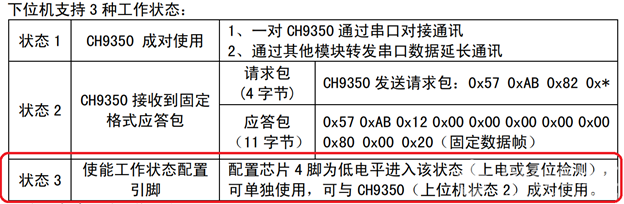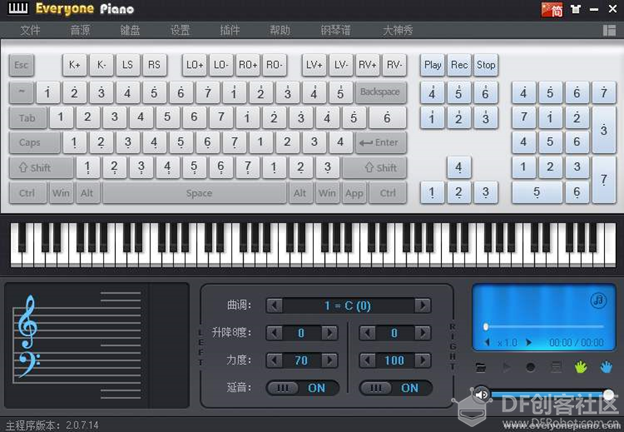``````#include <SoftwareSerial.h>
#include <SPI.h>
#include <MusicPlayer.h>

MusicPlayer player;

//实例化软串口,连接到 Music Shield
SoftwareSerial USBKB(2, 3); // RX, TX

byte KeyToNote(byte key)
{
byte v=0;
switch (key) {
case 0x1D: //Z
v=36;
break;
case 0x1B: //X
v=38;
break;
case 0x06: //C
v=40;
break;
case 0x19: //V
v=41;
break;
case 0x05: //B
v=43;
break;
case 0x11: //N
v=45;
break;
case 0x10: //M
v=47;
break;
case 0x36: //,
v=48;
break;
case 0x37: //.
v=50;
break;
case 0x38: // /
v=52;
break;

case 0x04: //A
v=48;
break;
case 0x16: //S
v=50;
break;
case 0x07: //D
v=52;
break;
case 0x09: //F
v=53;
break;
case 0x0A: //G
v=55;
break;
case 0x0B: //H
v=57;
break;
case 0x0D: //J
v=59;
break;
case 0x0E: //K
v=60;
break;
case 0x0F: //L
v=62;
break;
case 0x33: //;
v=64;
break;
case 0x34: //'
v=65;
break;

case 0x14:  //Q
v=60;
break;
case 0x1A: //W
v=62;
break;
case 0x08:  //E
v=64;
break;
case 0x15: //R
v=65;
break;
case 0x17: //T
v=67;
break;
case 0x1C: //Y
v=69;
break;
case 0x18: //U
v=71;
break;
case 0x0C: //I
v=72;
break;
case 0x12: //O
v=74;
break;
case 0x13: //P
v=76;
break;
case 0x2F: //[
v=77;
break;
case 0x30: //]
v=79;
break;

case 0x1E:  //1
v=72;
break;
case 0x1F: //2
v=74;
break;
case 0x20:  //3
v=76;
break;
case 0x21: //4
v=77;
break;
case 0x22: //5
v=79;
break;
case 0x23: //6
v=81;
break;
case 0x24: //7
v=83;
break;
case 0x25: //8
v=84;
break;
case 0x26: //9
v=86;
break;
case 0x27: //0
v=88;
break;
case 0x28: //-
v=89;
break;
case 0x29: //=
v=91;
break;
default:
break;
}
return v;
}
void setup()
{
//用于Arduino Debug 串口
Serial.begin(115200);

//用于和CH3995通讯
USBKB.begin(38400);

//初始化 Midi 功能
player.beginMidi();

delay(2000);

//设置主音量为最大
player.midiWriteData(0xB0, 0x07, 127);

//player.midiWriteData(0xB0, 0, 0x00);    //Default bank GM1

player.midiWriteData(0xC0, 1, 0);
}

//每笔 CH9350 键盘数据长度
#define CH9350KBLENGTH  11

//存放上一次 CH9350 发送过来的数据
char Last[CH9350KBLENGTH]={0,0,0,0,0,0,0,0,0,0,0};
//存放当前 CH9350 发送过来的数据
char Current[CH9350KBLENGTH];

byte i;
boolean Found;
void loop()
{
byte Index=0;

//取得 CH9350 送过来的当前按键信息
while (Index<CH9350KBLENGTH-1) {//不保存首位的 0x57 帧头，所以总共只有
while (Index<CH9350KBLENGTH-1) {
if (USBKB.available()) {
}
}
}
}

for (int p=0;p<CH9350KBLENGTH;p++) {Serial.print((byte)Current[p],HEX);Serial.print(" "); }
Serial.print("\n");

//检查 Current[Index],如果Last[]没有的，那么需要发送出去
for (Index=4;Index<CH9350KBLENGTH;Index++) {
Found=false;
if (Current[Index]!=0) {
for (i=4;i<CH9350KBLENGTH;i++) {
if (Current[Index]==Last[i]) {
Found=true;
}
}
if (Found==false) {
//Set instrument number. 0xC0 is a 1 data byte command
player.midiNoteOn(0, KeyToNote(Current[Index]), 127);
Serial.print("On ");
Serial.println(Current[Index],HEX);
Serial.print(" >> ");
Serial.println(KeyToNote(Current[Index]));
}
}
}

//检查 Last[Index],如果Current[]里面没有,那么需要发送NoteOff命令
for (Index=4;Index<CH9350KBLENGTH;Index++) {
Found=false;
if (Last[Index]!=0) {
for (i=4;i<CH9350KBLENGTH;i++) {
if (Last[Index]==Current[i]) {
Found=true;
}
}
if (Found==false) {
player.midiNoteOff(0, KeyToNote(Current[Index]), 127);
Serial.print("Off ");
Serial.println(KeyToNote(Last[Index]),HEX);
}
}
}

for (Index=0;Index<10;Index++) {Last[Index]=Current[Index];}
}``````

VS1053B 用来播放 MIDI 是很好的选择，这次使用的是微雪出品的 Music Shield 价格在 90 左右，淘宝最低价格有 35 的，我觉得应该同样能够使用。上海创客嘉年华